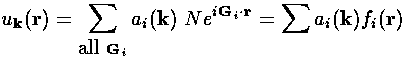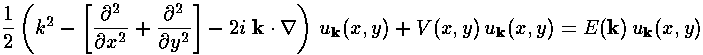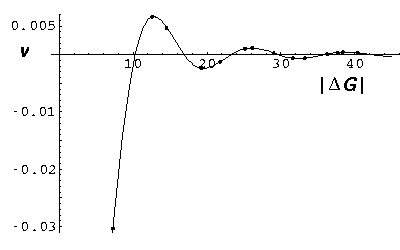## Band Structure p.9

The previously discussed grid approximation method has been introduced because I believe it to be understandable. It is not particularly computationally efficient, nor is it similar to methods actually used for real band structure calculation. Most approaches to band structure calculation involve plane wave expansion of the wavefunction. Although it is not the aim of these pages to introduce particularly innovative methods, I thought I should at least go one step down the plane wave expansion road, so you can begin to see a bit of what is required. I should also mention that our sample problem is designed to avoid many of the traps inherent in a realistic lattice models. Real approximation methods must focus on the very strong nuclear potential with multi-node core bound states that play little role in the electrical properties, but make for much more complex wavefunctions.

As stated before, a periodic function like uk can be expanded as a sum of reciprocal lattice vector waves like:

N exp(iG·r) = fi

where G is a reciprocal lattice vector and N=1/(a2 sin 60°)½ is a normalizing constant that forces the integral over a real lattice cell to be 1. (a2 sin 60° is the area of a lattice cell; the square-magnitude of the exponential part is 1.) The result is a Fourier series:Now uk must satisfy the Schrödinger's equation:which we write simply as:

H uk = E(k) uk

We now do the usual Fourier trick to isolate a particular expansion coefficient ai. We multiply both sides of the Schrödinger's equation by fi* and integrate over a lattice cell...orthonormality of the fi reduces the sum on the rhs to a single term; on the lhs we have a sum over all possible reciprocal lattice vectors labeled by j.< i | H | j > aj = E ai or

[H]·[a] = E [a]

where:Notice that we diagonalize the kinetic energy part of the Hamiltonian at the expense of spreading V--which was diagonal in the grid approximation--all over the matrix. Mathematica (or knowing a bit about special functions) allows us to express the V integral in terms of Bessel functions: J.

So far all is exact: instead of solving Schrödinger's differential equation we are now requested to solve an infinite-sized matrix eigenproblem. Our approximation consists of truncating the infinite matrix, thus including only a finite number of reciprocal lattice vectors (a.k.a., Fourier components). We justify this by noting that in the limit of "small" V0, the solutions are single waves and that the V matrix elements grow small for larger G differences...we say V causes only a small coupling of distant G. On the other hand, as V0 grows large, large G will be required to localize the ground state to a small region in space. Usually the only way to see if the truncation is a good approximation is to expand the number of included Fourier components and check to see that little is affected.

Below is a plot of the V matrix elements as a function of the magnitude of difference in G vector. The dots show the G vector differences in the actual reciprocal lattice. Notice that the diagonal V matrix elements (which are much larger: about -0.1) are not plotted since they produce only a uniform energy shiftWe now compare the results of band structure calculations using the 37 reciprocal lattice vectors within 3× the nearest neighbor distance (i.e., a 37×37 matrix eigenproblem); to the h=1/16 grid (which produces a 256×256 matrix eigenproblem). In the below plots the red lines are the new plane-wave approximation method and the black lines are the grid approximation. They agree closely, but the 37×37 matrix eigenproblem is solved about ten times faster.

### V0=16### V0=64### V0=256